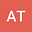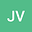Ultra-Compact accurate wave functions for He-like and Li-like iso-electronic sequences and variational calculus. II. Spin-singlet (excited) and spin-triplet (lowest) states of Helium sequence
•••• +1Juan Carlos Lopez Vieyra
UNAM
Author ProfileJuan Carlos del Valle
Universidad Nacional Autonoma de Mexico Instituto de Ciencias Nucleares
Author ProfileAuthor Profile## Abstract

As a continuation of Part I \cite{Part-1:2020} (Int. Journal of Quantum Chem. 2021; 121: qua.26586), dedicated to the ground state of He-like and Li-like isoelectronic sequences for nuclear charges $$Z\leq 20$$, a few ultra-compact wave functions in the form of generalized Hylleraas-Kinoshita functions are constructed, which describe the domain of applicability of the Quantum Mechanics of Coulomb Charges (QMCC) for energies (4-5 significant digits (s.d.)) of two excited states of He-like ions: the spin-singlet (first) excited state $$2^{1}S$$ and for lowest spin-triplet $$1^{3}S$$ state. For both states it provides absolute accuracy for energy $$\sim 10^{-3}$$ a.u., exact values for cusp parameters and also for 6 expectation values the relative accuracy $$\sim 10^{-2}$$. Bressanini-Reynolds observation about the special form of nodal surface of $$2^{1}S$$ state for Helium is confirmed and extended to ions with $$Z>2$$. Critical charges $$Z=Z_{B}$$, where ultra-compact trial functions loose their square-integrability, are estimated: $$Z_{B}(1^{1}S)\approx Z_{B}(2^{1}S)\sim 0.905$$ and $$Z_{B}(1^{3}S)\sim 0.902$$. For both states the Majorana formula - the energy as the second degree polynomial in $$Z$$ - provides accurately the 4-5 significant digits for $$Z\leq 20$$.

20 Aug 2021Submitted to International Journal of Quantum Chemistry
20 Aug 2021Submission Checks Completed
20 Aug 2021Assigned to Editor
02 Sep 2021Reviewer(s) Assigned
18 Sep 2021Review(s) Completed, Editorial Evaluation Pending
21 Sep 2021Editorial Decision: Revise Major